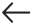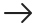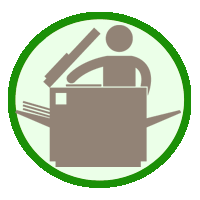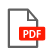CLOSE

# Un peu de tout Mathématiques 5These documents allow us to have at hand various exercises based on math programs. Included is counting, odd and even numbers, square roots, Roman numerals, time, measurement, geometry, fractions, numbers with commas, addition, subtraction, multiplication, division, math problems, etc.

• Author: Stéphan Galibois
• Codes :
Paper Document - 7152
PDF Document - 7152PDF
• Reproduction:• Digital Version: